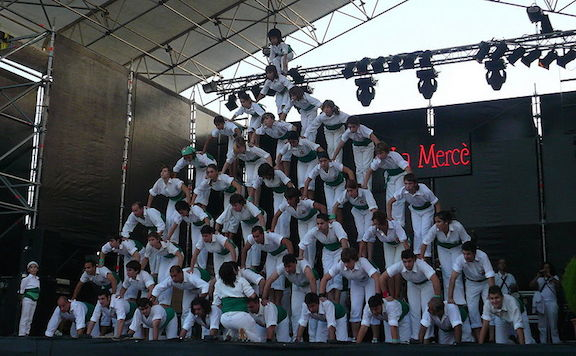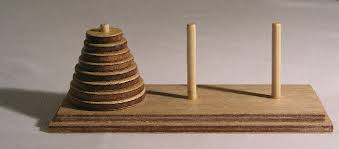Probability

# Introduction to Recursion: Level 4 Challenges

A toy model for a growing network is the recursive tree. The tree starts as a single node and at each step in the growth process, one new node is added which makes a single connection, at random, to an existing node.

Assume that the network has grown large enough so that its statistical properties are effectively constant. The fractions of nodes that have connections to exactly 7 other nodes is given by some fraction $\dfrac ab$, where $a$ and $b$ are positive coprime integers. What is the value of $a + b$?A human pyramid is built up by several layers. At the top, there is one person, and each subsequent layer has 1 additional person. Each person is supported by the 2 people that are beneath them.

Consider a 9 layer pyramid, which comprises 45 people. Assuming that all the cheerleaders weigh 128 pounds each, and that the weight is equally distributed amongst both supporters, what is the most weight supported by anyone in this pyramid?

How would you generalize this?

Image credit: Wikipedia Pere Lopez

How many ways can the numbers $1,2,3,4,5,6,7$ be arranged in a row such that the numbers in the 2nd, 4th, and 6th positions are each larger than both of their neighbours?The Tower of Hanoi is a traditional game where there are 3 pegs and $n$ discs arranged as seen in picture. All discs are to be moved from $1^{st}$ peg $P_1$ to $3^{rd}$ peg $P_3$ such that only one disc is moved at once. But no disc should be kept on one smaller than it during the process. So the minimum number of moves required to transfer all discs to $P_3$ (say $a_n$) is counted by $a_n=2a_{n-1}+1$ and $a_1=1$ i.e. after solving becomes $a_n=2^n-1.$

If we have a Tower of Hanoi with 42 pegs, and we have an additional rule that no disc can be moved more than twice, what is the maximum number of discs such that all discs can be moved to pegs other than the first peg $P_1?$A single snowflake rests at the origin, $(0, 0)$, on an infinite cartesian plane. At time $t = 0$, it divides and replicates itself, moving exactly $1$ unit in each of the $8$ major compass directions (N, NE, E, SE, S, SW, W, and NW), leaving $(0, 0)$ vacant.

At $t = 1$, all snowflakes which occupy the exact same points are merged into one, and then the process repeats for every snowflake, resulting in the following pattern:So, to iterate the first few steps:

• There is $1$ snowflake at time $t = 0$
• At time $t = 1$, there are $8$ snowflakes pre-merge.
• No snowflakes coincide, so there are $8$ snowflakes post-merge as well.
• At time $t = 2$, there are $64$ snowflakes pre-merge.
• Post-merge, only $33$ snowflakes remain.

How many snowflakes are there at time $t = 1000$, post-merge?

×